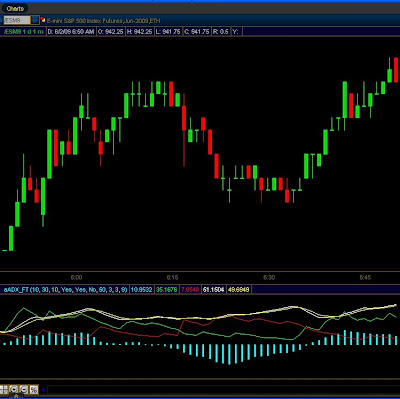## Tuesday, June 2, 2009

### Code example for referencing TOS studies for a readers emailA reader wrote in and asked a question. I'm not sure if I understood exactly what my reader wanted to do but here is one example of leveraging TOS studies.

Reference SimpleMovingAvg(length=21). That would return the SMA value for that bar. If the SMA(21) was less than the close of that bar it would print a point at the specified value.

insideBar.setHiding( if(close > reference SimpleMovingAvg(length=21),1,0));

So if(close > reference SimpleMovingAvg(length=21),1,0)) says that if close > SMA(21) then return true, else return false. Return value true activates the Hide function, return value 0 does not activate it.

(Thanks for the email. I hope you don't mind me sharing a portion of it so all can learn. Best regards, Freethinkscript).

input offset = 1 ;
plot InsideBar = if close < close then high + offset else double.NaN;

insideBar.SetPaintingStrategy(paintingStrategy.POINTS);
InsideBar.SetDefaultColor(color.LIGHT_GREEN);
insideBar.SetLineWeight(5);
insideBar.setHiding( if(close > reference SimpleMovingAvg(length=21),1,0));Developed by Dr. Martin Zweig, the Breadth Thrust Indicator measures market momentum. The Breadth Thrust is calculated by dividing a 10-day exponential moving average of the number of advancing issues, by the number of advancing plus declining issues.

A "Breadth Thrust" occurs when, during a 10-day period, the Breadth Thrust indicator rises from below 40% to above 61.5%. A "Thrust" indicates that the stock market has rapidly changed from an oversold condition to one of strength, but has not yet become overbought. According to Dr. Zweig, there have only been fourteen Breadth Thrusts since 1945. The average gain following these fourteen Thrusts was 24.6% in an average time-frame of eleven months. Dr. Zweig also points out that most bull markets begin with a Breadth Thrust.

A weekly chart is best for this indicator.declare lower;

plot zw = ExpAverage(data = close("\$advn"), length = 10 ) /( ExpAverage(data=close("\$advn"), length=10) + ExpAverage(data=close("\$decn"), length = 10));
plot forty = 0.40;
forty.SetDefaultColor(Color.RED);
plot sixfifteen = 0.615;
sixfifteen.SetDefaultColor(Color.Yellow);

### ADX, DMI, MACD tri-indicator for one low price - FREEMany times a combination of indicators can shead more light on price movement. Try this combination and see how you like it. Change the input value invertNegMACD = Yes to see a more visual picture of MACD.

You can see ADX is colored White, and also ADX Average is colored Yellow. DMI(positive) is Green, DMI(Negative) is Red, and MACD is painted as a Histogram.declare lower;
input MACDfastLen = 10;
input MACDslowLen = 30;
input MACDLen = 10;
input showADX_DMI = { "No", default "Yes"};
input showMACD = { "No", default "Yes"};
input invertNegMACD = { "Yes", default "No"};
input MACDHeight = 50;
input MACDWidth = 3;
input DMI_Len = 9;

def fastAvg = Ema2(data = close, "smoothing factor" = 2 / (1 + MACDfastLen));
def slowAvg = Ema2(data = close, "smoothing factor" = 2 / (1 + MACDslowLen));
def Value = fastAvg - slowAvg;
def nextAvg = ExpAverage(data = Value, MACDLen);
def HistoBar = value - nextAvg;
def HiScale = HighestAll(HistoBar);
def LoScale = AbsValue(LowestAll(HistoBar));
def BarScale = if HiScale > LoScale then HiScale else LoScale;

plot macd_plot = if (showMACD, if( invertNegMACD, if ( HistoBar < 0,( -1 * HistoBar * MACDHeight / BarScale ),( HistoBar * MACDHeight / BarScale )),HistoBar * MACDHeight / BarScale), double.nan);

macd_plot.AssignValueColor(if invertNegMACD then if HistoBar >= 0 then Color.Cyan else Color.Magenta else Color.Cyan);
macd_plot.SetPaintingStrategy(PaintingStrategy.Histogram);
macd_plot.SetLineWeight(MACDWidth);

def hiDiff = high - high;
def loDiff = low - low;
def plusDM = if hiDiff > loDiff and hiDiff > 0 then hiDiff else 0;
def minusDM = if loDiff > hiDiff and loDiff > 0 then loDiff else 0;
def ATR = WildersAverage(TrueRange(high, close, low), DMI_Len);
plot "DI+" =
if showADX_DMI then 100 * WildersAverage(plusDM, DMI_Len) / ATR
else double.nan;
plot "DI-" =
if showADX_DMI then 100 * WildersAverage(minusDM, DMI_Len) / ATR
else double.nan;
def DX =
if ("DI+" + "DI-" > 0) then 100 * AbsValue("DI+" - "DI-") / ("DI+" + "DI-")
else 0;

"DI+".SetDefaultColor(Color.Green);
"DI-".SetDefaultColor(Color.Red);

## Monday, June 1, 2009

### Keeping an eye on Volume Weighted MACDMACD measures the difference between two Exponential Moving Averages (EMAs). A positive MACD indicates that the 12-day EMA is trading above the 26-day EMA. On the other hand, a negative MACD indicates that the 12-day EMA is trading below the 26-day EMA. Basically, when the MACD is above the 0 line, it is indicating that the momentum is bullish. When the MACD is negative however, it is indicating that the momentum is bearish.

When the 12 days moving average crosses the 26 day moving average, you should watch the next price movements very carefully as it tends to signal a trend reversal. If the 12 days MA crosses above the 26 days MA, it’s a bullish signal. On the flip side, if the 12 days MA crosses below the 26 das MA, it’s a bearish signal.This is a good indicator to watch while markets are moving like they are now.

declare lower;

input fastLength = 12;
input slowLength = 26;
input MACDLength = 9;

def fastAvg = sum(data = (close * volume), length = fastLength)/sum(data = volume, length = fastLength);

def slowAvg = sum(data = (close * volume), length = slowLength)/sum(data = volume, length = slowLength);

plot Value = fastAvg - slowAvg;
plot Avg = ExpAverage(data = Value, length = MACDLength);

plot baseline = 0;
plot diff = value - avg;
diff.AssignValueColor (if diff >= 0 then Color.UPTICK else Color.DOWNTICK);
diff.SetPaintingStrategy(PaintingStrategy.HISTOGRAM);

diff.SetDefaultColor(GetColor(8));
Avg.SetDefaultColor(GetColor(5));
Value.SetDefaultColor(GetColor(1));
baseline.SetDefaultColor(GetColor(0));

(can also be found in default studies)